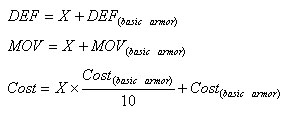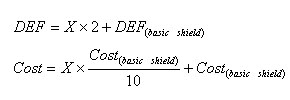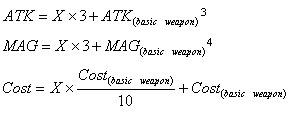Site Navigation•RPGClassics Main•Contact Maintainers:•Skankin Garbage•WalhallaShrine Navigation•Shrine Home•Antz Colony•Armor•Bestiary•Bosses•Characters•D-Ratio•General Information•Items•Kokon-Horay•Neat Tricks n' Stuff•Shields•Shopping List•Skills•SOL•Soundtrack•Thanks•Walkthrough•Weapons

You Definitely Are A Nerd
(Hah, like you didn't see that one coming)

Uh, hi. Un/Fortunately, this page isn't a joke. :D I'll spend the next few paragraphs explaining the mathematical relations behind the "+X" stuff next to armor, shields and weapons because I KNOW you've always wondered about its true meaning. And quite frankly, it's better to explain how it works than trying to list the location of all the "+X" equipment. :P

Firstly, you should know by now that the selling price of everything (armor, items, shields, skills and weapons) is equal to the buying price divided by 4. Secondly, all variables influenced by the "+X" thing follow a linear equation of the type "y = mX + b". Thirdly, if you have no idea of what I'm talking about, don't worry. It won't change your way of playing through the game. :P Fourthly, and last, you should definitely check this page if you're like "Woah dude, linear equations? Cool!"

 Armor Shields Weapons More!

Armor 1

The "X" value has an effect on the DEF, MOV and cost of the corresponding armor. The equations are as follows :Aww, I even wasted my time to present the equations in a beautiful and professional format. Anyway, let's use the Ranger Suit as an example. It has an initial DEF of 14, MOV of 10 and cost of 360z. So, the Ranger Suit+2 would have a DEF of 16 (2 + 14 = 16), MOV of 12 (2 + 10 = 12) and cost of 432z (2 x (360/10) + 360 = 432).

1 As in all good grammatical mathematical rules, there's always at least an exception : Nina's Plasma Check doesn't exactly follow the cost relation. ...Did it change your life? Yeah, I knew it would.

Shields

The "X" value has an effect on the DEF and cost of the corresponding shield. Here are the equations :Let's use the Ranger Shell as an example. It has an initial DEF of 3 and cost of 320z. So, the Ranger Shell+4 would have a DEF of 11 (4 x 2 + 3 = 11) and cost of 448z (4 x (320/10) + 320 = 448).

Weapons 2

The "X" value has an effect on the ATK, MAG and cost of the corresponding weapon. Below are the equations :Let's use the Ranger Edge as an example. It has an initial ATK of 16, MAG of "---" and cost of 400z. So, the Ranger Edge+1 would have an ATK of 19 (1 x 3 + 16 = 19), MAG of 0 (or "---", if you prefer) and cost of 440z (1 x (400/10) + 400 = 440).

2 Ryu's Strong Sword doesn't exactly follow the relations, but hey, it's close enough.
3 This equation applies only for weapons that have attack points. Check the Weapons page to see which ones do and which don't.
4 This equation applies only for weapons that have magical properties. Maybe you should check the Weapons page again to see which ones do and which don't. And I like to write subnotes because it makes me look so cool and smart.